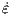## List of Symbols

### Overview of Solid Mechanics and Finite Element Method

 Xi Lagrangian Cartesian Coordinates, i=1,2,3 xi Eulerian Cartesian Coordinates, i=1,2,3 u Displacement Field lij Lagrange Infinitesimal Strain Tensor εij,ε Eulerian Infinitesimal Strain Tensor t Stress Vector σ Cauchy Stress Tensor τij Shear Stress Components F Body Force b Force per Unit of Mass ρ Density of a Body σmises Von Mises Effective Stress εe Elastic Strain Tensor ε p Plastic Strain Tensor C Elasticity Tensor M Compliance Tensor λ Lame´ Constant E Young’s Modulus ν Poisson’s Ratio μ Shear Modulus α Coefficient of Thermal Expansion δu Virtual Displacement δεij Virtual Strain Components

### Stress Evolution during 3D IC Stacking using Open TSVs

 pm Pressure of an Indenter Y Uniaxial Compressive Yield Stress of a Sample a Contact Area between Indenter and Sample E* Reduced Modulus R Radius of an Indenter P Applied Load by Indenter d Penetration Depth in a Sample Qp Plastic Potential I1 First Invariant of Cauchy Stress Tensor J2 Second Invariant of Cauchy Stress Tensor J3 Third Invariant of Cauchy Stress Tensorp Plastic Strain Tensor Incrementpe Effective Plastic Strain Rate Fy Yield Function σys0 Initial Yield Stress ETiso Isotropic Tangent Modulus

### Fracture Mechanics and Delamination in Open TSV

 ZI Westergaard Function KI,KII,KIII Stress Intensity Factors for mode I, II, and III, respectively Φ Airy Stress Function ε Bimaterial Constant G Energy Release Rate Gc Critical Energy Release Rate Π Total Potential Energy U Total Strain Energy W Strain Energy Density V Potential of External Force γ Fracture Surface Energy J J-Integral

### Simulation of Intrinsic Stress Build-Up in Thin Metal Films

 R Radius Parameter Ri Initial Radius of the Island Rfr Frozen-In Radius hf Film Thickness f Surface Stress ΔP Laplace Pressure γs Surface Energy γgb Grain Boundary Energy hgb Grain Boundary Height vg Deposition Rate vgb Grain Boundary Velocity μs Surface Chemical Potential μgb Grain Boundary Chemical Potential Ngb Number of Atoms Moving from the Surface to Grain Boundary D Atom Diffusivity a Height of an Atomic Step Cs Fractional Coverage of Adatoms σT Tensile Stress σC Compressive Stress Ω Atomic Volume r Island Radius at the Coalescence Point L Grain Size ws Width of the Scallops hs Height of the Scallops